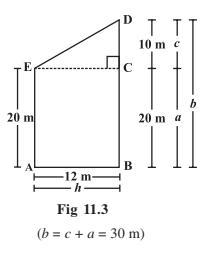# Mensuration Class 8

Mensuration class 8 Chapter 11 important questions and formulas are mentioned here. Get through the formulas and practice the concept of mensuration with the help of given examples. This chapter has been further introduced in Class 10, hence it is necessary that each student learns its basic concept first. Mensuration basically means the measurement of area and perimeter of different geometrical shapes such as triangle, trapezium, rectangle, etc. Preparing this chapter from this article will help students in scoring good marks in the final exams.

## Mensuration Class 8 Extra Questions

Here are some questions provided for Maths chapter 11 of 8th standard, Mensuration. Go through these questions and practice more problems based on this concept.

By constructing EC || AB, we can split the given figure (AEDCBA) into two parts (Triangle ECD right-angled at C and Rectangle AECB), Here, b = a + c = 30 mNow, Area of Triangle DCE:

1/2 × CD × EC= 1/2 × c × s

h = 1/2 ×10× 12 = 60 m2

Also, Area of rectangle AECB = AB × BC = h × a=12 × 20=240 m2

Therefore, Area of trapezium AEDB = Area of Triangle DCE + Area of rectangle AECB = 60 + 240 = 300 =300m2Diagonal AC divides the given quadrilateral into two triangles i.e. Triangle ABC and Triangle ADC.

Now, Area of Quadrilateral ABCD = Area of Triangle ABC + Area of Triangle ADC.

=1/2 × AC × h1 + 1/2 × AC × h2 =1/2 × d × (h1 +h2)

Where, d = The length of diagonal of a quadrilateral.

### Mensuration Class 8 Formulas and Notes

The important formulas covered in this chapter are as follows:

 Area of Trapezium height x (sum of parallel sides)/2 Area of Rhombus ½ x d1 x d2 Area of Special Quadrilateral ½ x d x (h1 + h2) Surface area of Cuboid 2(lb x bh x hl) Surface area of Cube 6a2 Surface area of cylinder 2πr(r + h) Volume of Cuboid l × b × h Volume of Cube a3 Volume of cylinder πr2h

Where,

• d1 and d2 are the diagonals of the rhombus
• d is the diagonal of a special quadrilateral which is divided into two triangles
• h1 and hare the two perpendiculars from the vertices of a quadrilateral to its diagonal
• l, b and h denotes length, breadth and height of the cuboid
• r represents the radius of the cylindrical base
• a is the side of the cube

Note:

• The trapezium and rhombus are the figures represented in the two-dimensional plane. Whereas Cuboid, Cube and Cylinder are three-dimensional solid shape.
• The surface area of a solid shape is the sum of the areas of its faces.
• Amount of region occupied by a solid shape is called its volume.

## Solved Questions

Example 1: Find the area of a square whose side is 30 m.

Solution: The area of square = (Side)2

Given, side = 30 m.

Then, area of square = (30)2 = 900 m2.

Example 2: Find the perimeter of a triangle with two equal sides of 5 cm and one side of 10 cm.

Solution: We know that,

perimeter of triangle = sum of all the three sides

= (5 + 5 + 10)

= 20 cm.

Example 3: Calculate the area of a trapezium whose height is 4 cm and length of parallel sides are 5 cm and 3 cm respectively.
Solution: Given, Height of the trapezium = 4cm and length of parallel sides are 5cm and 3cm respectively.

Area of trapezium = height x (sum of parallel sides)/2

A = 4 x (5 + 3)/2

A = 4 x 8/2

A = 4 x 4

A = 16 sq.cm.

Example 4: Find the area of special quadrilateral whose diagonal length is 6 cm. And the lengths of two perpendiculars on given diagonal from the vertices are 3 cm and 4 cm.

Solution: Given, diagonal of quadrilateral, d = 6 cm.

Let the length of two perpendiculars be h1 = 3 cm and h2 = 4 cm.

As per the formula of quadrilateral, we can write,

Area of quadrilateral ABCD =  ½ x d x (h1 + h2)

A = 1/2 x 6 x (3 + 4)

= 1/2 x 6 x 7

= 3 x 7

= 21 sq.cm

Example 5: Find the area of rhombus in which the length of its two diagonals is 8.5cm and 10 cm.

Solution: We know;

Area of rhombus = ½ x d1 x d2

A = 1/2 x 8.5 x 10

A = 42.5 sq.cm.

Test your knowledge on Mensuration Class 8